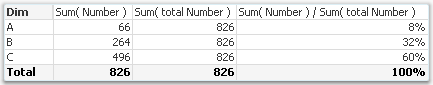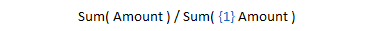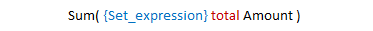# Qlik Design Blog

All about product and Qlik solutions: scripting, data modeling, visual design, extensions, best practices, etc.Employee

## The Aggregation Scope

When a calculation is made in a QlikView session, it always involves an aggregation over the relevant data records. But which records are relevant? What is the scope of the aggregation?

This seems like a simple question, but there are in fact quite a few things that could be said about it.

Normally, there are two different restrictions that together determine which records are relevant: The Selection, and – if the formula is found in a chart – the Dimensional value. The aggregation scope is what remains after both these restrictions have been taken into consideration.

But not always…

There are ways to define your own aggregation scope: This is needed in advanced calculations where you want the aggregation to disregard one of the two restrictions. A very common case is when you want to calculate a ratio between a chosen number and the corresponding total number, i.e. a relative share of something.In other words: If you use the total qualifier inside your aggregation function, you have redefined the aggregation scope. The denominator will disregard the dimensional value and calculate the sum of all possible values. So, the above formula will sum up to 100% in the chart.However, there is a second way to calculate percentages. Instead, you may want to disregard the the selection in order to make a comparison with all data before any selection. Then you should not use the total qualifier; you should instead use Set analysis:Using Set analysis, you will redefine the Selection scope. The set definition {1} denotes the set of all records in the document; hence the calculated percentages will be the ratio between the current selection and all data in the document, split up for the different dimensional values.In other words: by using the total qualifier and set analysis inside an aggregation function, you can re-define the aggregation scope.

• To disregard the dimensional grouping – Use the Total qualifier
• To disregard the selection – Use Set Analysis

The above cases are just the basic examples. The total qualifier can be qualified further to define a subset based on any combination of existing dimensions, and the Set analysis can be extended to specify not just “Current selection” and “All data”, but any possible selection.

And, of course the total qualifier can be combined with Set analysis.A final comment: If an aggregation is made in a place where there is no dimension (a gauge, text box, show condition, etc.), only the restriction by selection is made. But if it is made inside a chart or an Aggr() function, both restrictions are made. So in these places it could be relevant to use the total qualifier.

HIC

Further reading related to this topic:

What does the TOTAL qualifier do?

Totals in Charts

A Primer on Set Analysis